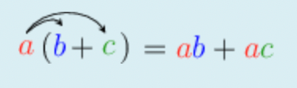Home Practice
For learners and parents For teachers and schools
Textbooks
Full catalogue
Pricing SupportLog in

We think you are located in United States. Is this correct?

# Test yourself now

High marks in science are the key to your success and future plans. Test yourself and learn more on Siyavula Practice.

## 8.6 Chapter summary

• The algebraic expressions that involve division are called algebraic fractions. The rules that govern operations with fractions also apply to algebraic fractions.
• Here are some important things to remember about fractions:
• When multiplying fractions, we multiply the numerators and multiply the denominators: $$\frac{a}{b} \times \frac{c}{d} = \frac{a \times c}{b \times d}$$. So, we can also go the opposite way to separate the fraction when we have a fraction with only products: $$\frac{a \times c}{b \times d} = \frac{a}{b} \times \frac{c}{d}$$
• When adding (or subtracting) fractions, if the denominators are the same, then we can add (or subtract) the numerators: $$\frac{a}{c} + \frac{b}{c} = \frac{a + b}{c}$$. So, we can also go the opposite way to separate the fraction when we have a fraction with addition (or subtraction) in the numerator: $$\frac{a + b}{c} = \frac{a}{c} + \frac{b}{c}$$.
• To simplify an expression:
• Separate it into terms. Terms are separated by addition and subtraction symbols, and joined into one by multiplication and division symbols, and brackets.
• Simplify each term (if needed).
• Add or subtract like terms from left to right (if possible).
• To simplify the algebraic expressions with roots:
• Square roots, e.g. $$\sqrt{16x^{2}}$$. Expand the power, then group the bases into two equal groups. $$\sqrt{16x^{2}} = \sqrt{(4x) \times (4x)} = \sqrt{(4x)^{2}} = 4x$$
• Cubic roots: e.g. $$\sqrt{t^{6}}$$. Expand the power, then group the bases into three equal groups. $$\sqrt{3t^{2} \times 3t^{2} \times 3t^{2}} = \sqrt{\left( 3t^{2} \right)^{3}} = 3t^{2}$$
• Recall the distributive property of multiplication over addition (or subtraction):• The process of writing product expressions as sum expressions is called expansion. It is sometimes also referred to as multiplication of algebraic expressions.